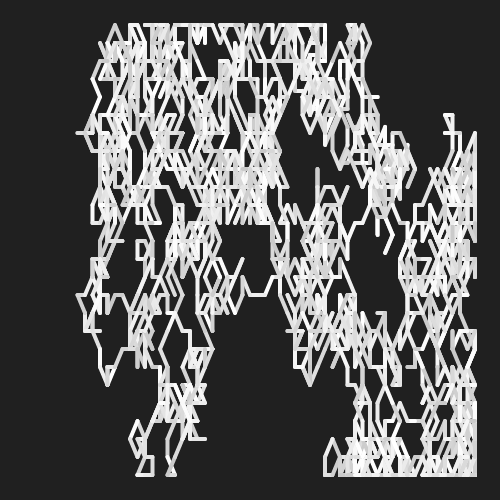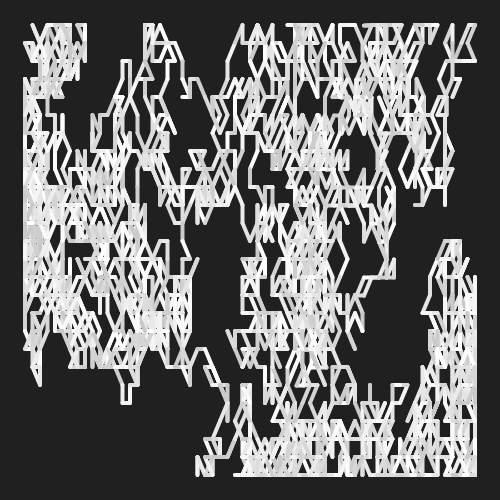# Random Notes

example p5.js javascript libraries sound genuary

Note: This sounds pretty bad on Android, and sometimes you have to click to hear anything. Coding is fun!

``````const minNote = 250;
const maxNote = 500;
const minSpeed = 2;
const maxSpeed = 8;
const margin = 25;

let monoSynth;
let note;
let speed;

let prevNote;
let prevSpeed;

function setup() {
createCanvas(400, 400);
monoSynth = new p5.MonoSynth();

note = random(minNote, maxNote);
speed = random(minSpeed, maxSpeed);
prevNote = note;
prevSpeed = speed;

strokeWeight(4);
background(32);
}

function draw() {

const frameDivider = floor(60 / speed);

if (frameCount % frameDivider == 0) {
playSynth();
}
}

function playSynth() {
userStartAudio();

note += random([-10, 0, 10]);
note = constrain(note, minNote, maxNote);

speed += random([-.1, 0, .1]);
speed = constrain(speed, minSpeed, maxSpeed);

const velocity = 1;
const time = 0;
const duration = 1 / speed;

monoSynth.play(note, velocity, time, duration);

const prevSpeedX = map(prevSpeed,
minSpeed, maxSpeed,
margin, width - margin);
const prevNoteY = map(prevNote,
minNote, maxNote,
height - margin, margin);
const speedX = map(speed,
minSpeed, maxSpeed,
margin, width - margin);
const noteY = map(note,
minNote, maxNote,
height - margin, margin);

stroke(random(200, 255));
line(prevSpeedX, prevNoteY, speedX, noteY);
prevNote = note;
prevSpeed = speed;
}
``````

This sketch uses the p5.sound library to play random notes. It also draws a line based on the speed and the notes it plays, so you get a visualization of the random “song” it plays.

I created this for the 28th day of Genuary which had a prompt of “Use sound.”# Remix Ideas

• Create a different visualization for the random notes. Maybe lower notes become more red, or faster notes fade out.
• Explore the rest of the p5.sound library and try other classes and functions.
• Recreate a song using the library. Start with something like “Twinkle, Twinkle, Little Star” and work your way up to your favorite song!